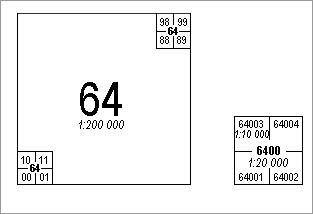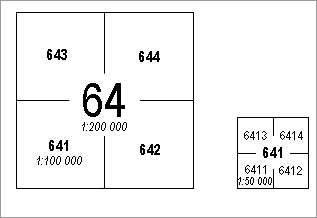# Map Sheet Indexes and Coordinate Systems

Map sheets are oriented in relation to the central meridian, the x-coordinate increases towards the north and the y-coordinate towards the east.

The sheet line system is based on the map sheet index at 1:200 000.

## 1:20 000 and 1:10 000 Map Sheets

The Estonian Basic Map is divided into 50x50 cm map sheets. At the scale of 1:10 000 the map sheet covers an area of 5x5 km, at the scale of 1:20 000 the area is 10x10 km.

By dividing the 1:200 000 map sheet into 100 smaller squares (numeration starting from the left bottom) we get a 1:20 000 Basic Map sheet.

The number of 1:20 000 Basic Map has four digits and consists of two parts:

1. 1:200 000 sheet number
2. 1:20 000 sheet number(00-99)

A dot is used between the two numbers, e.g.64.10Left is 1:20 000 and right is 1:10 000 map sheet number derivation

By dividing the 1:20 000 map sheet into four (numeration starting again from the left bottom) we get a 1:10 000 map sheet, the number of which has 5 digits (e.g.64.103) and consists of the following parts:

1. 1:200 000 sheet number
2. 1:20 000 sheet number(00-99)
3. 1:10 000 sheet number(1-4

## 1:100 000 and 1:50 000 Map Sheets

The 1:200 000 sheet is divided into four, the outcome being 1:100 000 sheet number which has three digits (e.g.641).

The 1:100 000 sheet is divided into four, which results in 1:50 000 map sheet number that has four digits (e.g. 6411).Left is 1:100 000 and right 1:50 000 map sheet number derivation

The Estonian Topographic Map 1:50 000 is divided into 50x50 cm map sheets. At the scale of 1:50 000 the map sheet covers an area of 25x25 km.

Unlike the 1:20 000 map sheet index the numbers are not separated by a dot.

## 1:2000 Map Sheets

1:2000 map sheets are also 50x50 cm squares that cover the area of 1x1 km.